Question

# Term Answer Description Risk A. The potential for variability in the possible outcomes associated with an...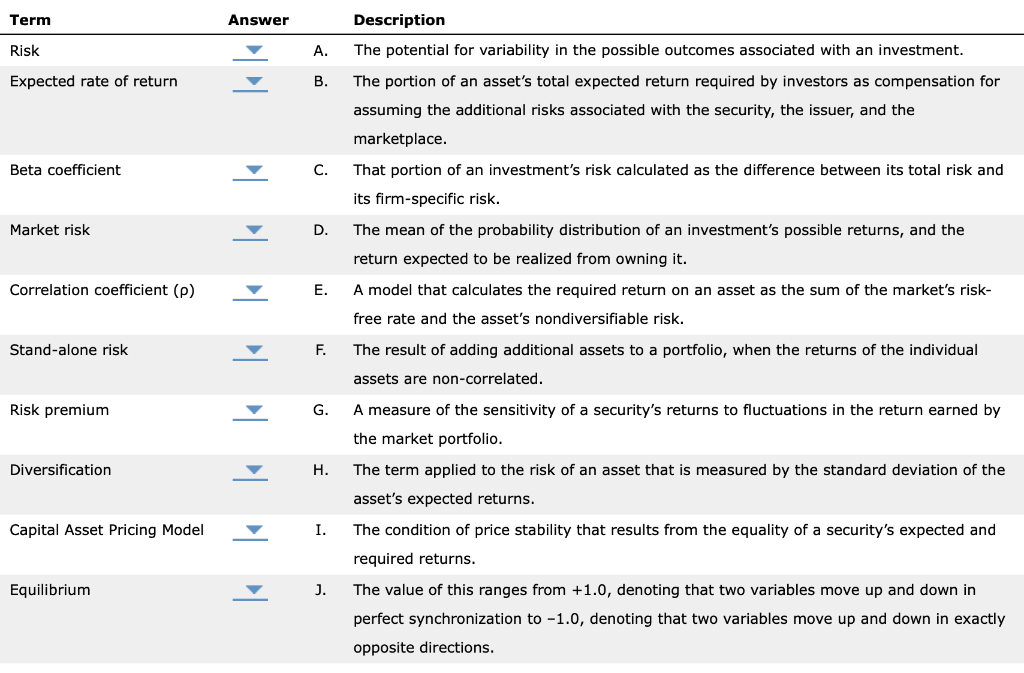Term Answer Description Risk A. The potential for variability in the possible outcomes associated with an investment. Expected rate of return The portion of an asset's total expected return required by investors as compensation for assuming the additional risks associated with the security, the issuer, and the marketplace. Beta coefficient That portion of an investment's risk calculated as the difference between its total risk and its firm-specific risk. Market risk The mean of the probability distribution of an investment's possible returns, and the return expected to be realized from owning it. Correlation coefficient (p) A model that calculates the required return on an asset as the sum of the market's risk- free rate and the asset's nondiversifiable risk. Stand-alone risk The result of adding additional assets to a portfolio, when the returns of the individual assets are non-correlated. A measure of the sensitivity of a security's returns to fluctuations in the return earned by the market portfolio. Risk premium o Diversification i The term applied to the risk of an asset that is measured by the standard deviation of the asset's expected returns. Capital Asset Pricing Model I. The condition of price stability that results from the equality of a security's expected and required returns. Equilibrium J. The value of this ranges from +1.0, denoting that two variables move up and down in perfect synchronization to -1.0, denoting that two variables move up and down in exactly opposite directions.

Correct match is as follows:

Risk -A

Expected rate of return -D

Beta -G

Market risk-C

Correlation Coefficient-J

Stand Alone risk-H

Diversification-F

CAPM-E

Equilibrium-I

#### Earn Coins

Coins can be redeemed for fabulous gifts.

Similar Homework Help Questions
• ### Term Answer Description Risk A. The risk of an asset when it is the only asset...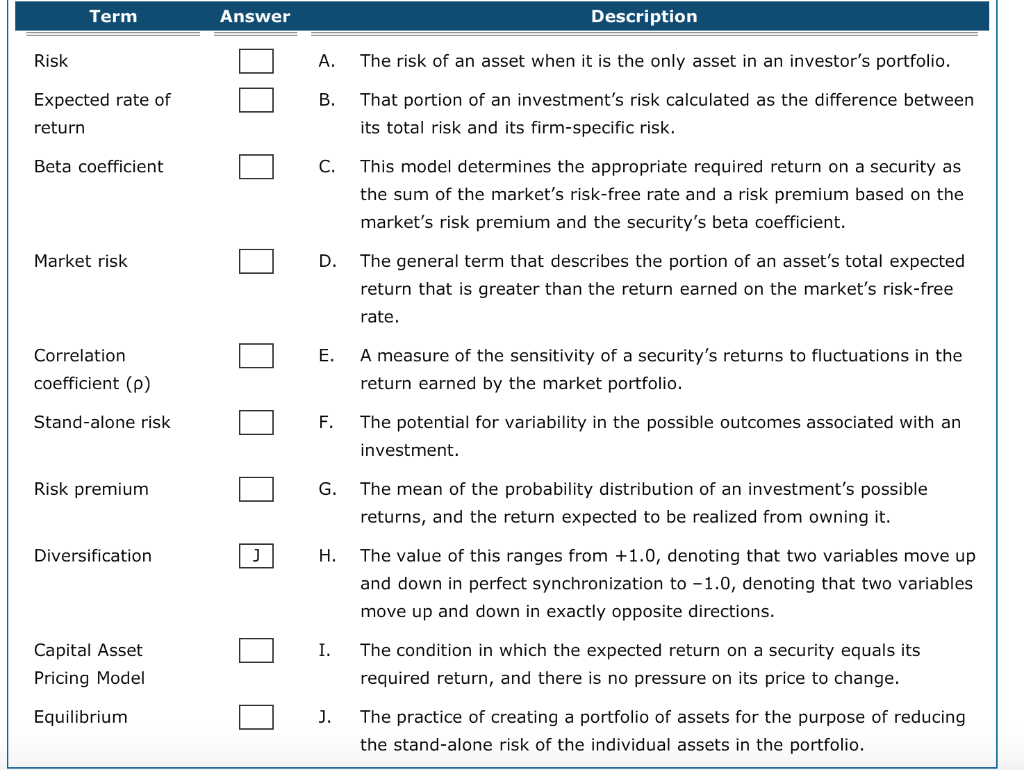Term Answer Description Risk A. The risk of an asset when it is the only asset in an investor's portfolio. Expected rate of return That portion of an investment's risk calculated as the difference between its total risk and its firm-specific risk. Beta coefficient This model determines the appropriate required return on a security as the sum of the market's risk-free rate and a risk premium based on the market's risk premium and the security's beta coefficient. Market risk The...

• ### Term Description Risk A. The term applied to the risk of an asset that is measured...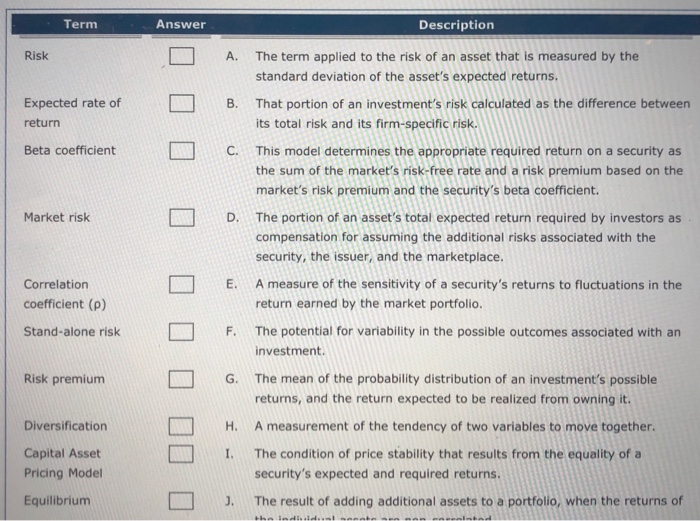Term Description Risk A. The term applied to the risk of an asset that is measured by the standard deviation of the asset's expected returns. That portion of an investment's risk calculated as the difference between its total risk and its firm-specific risk. Expected rate of return B. Beta coefficient L C . This model determines the appropriate required return on a security as the sum of the market's risk-free rate and a risk premium based on the market's risk...

• ### Term Answer Description A. The general term that describes the portion of an asset's total expected...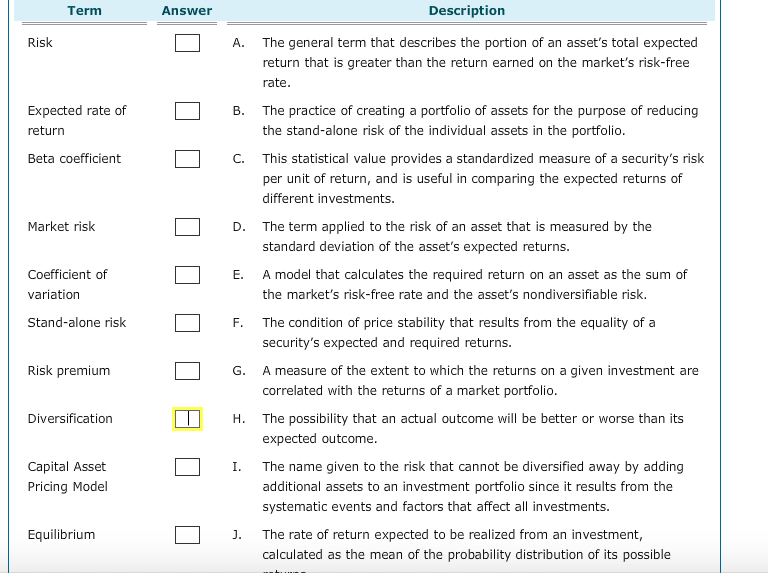Term Answer Description A. The general term that describes the portion of an asset's total expected return that is greater than the return earned on the market's risk-free rate Expected rate of return B. The practice of creating a portfolio of assets for the purpose of reducing the stand-alone risk of the individual assets in the portfolio Beta coefficient C. This statistical value provides a standardized measure of a security's risk per unit of return, and is useful in comparing...

• ### Ch 02: Assignment - Risk and Return: Part 1 Term Answer Risk A Expected rate of...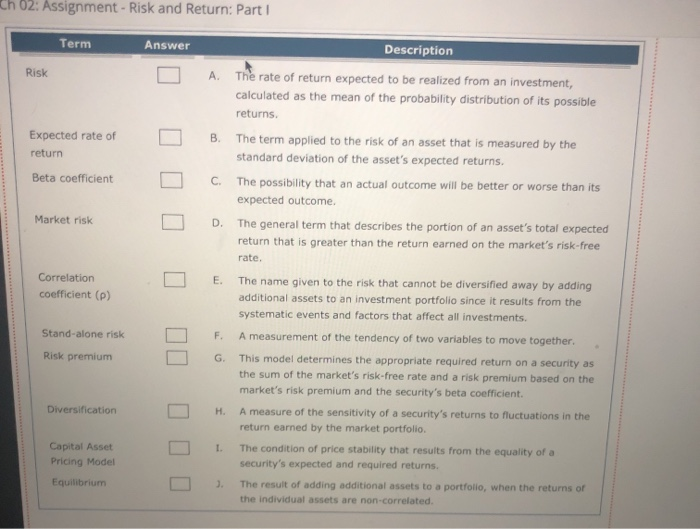Ch 02: Assignment - Risk and Return: Part 1 Term Answer Risk A Expected rate of return B Description The rate of return expected to be realized from an investment, calculated as the mean of the probability distribution of its possible returns. The term applied to the risk of an asset that is measured by the standard deviation of the asset's expected returns. The possibility that an actual outcome will be better or worse than its expected outcome The general...

• ### 2. 3: Risk and Rates of Return: Risk in Portfolio Context Risk and Rates of Return:...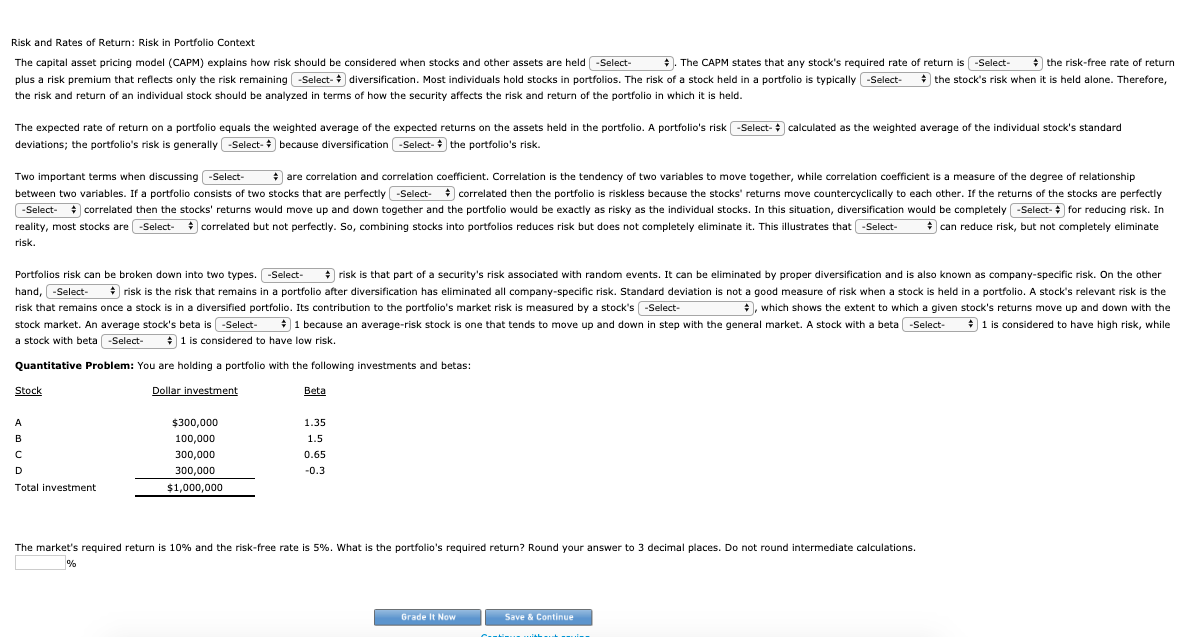2. 3: Risk and Rates of Return: Risk in Portfolio Context Risk and Rates of Return: Risk in Portfolio Context The capital asset pricing model (CAPM) explains how risk should be considered when stocks and other assets are held . The CAPM states that any stock's required rate of return is the risk-free rate of return plus a risk premium that reflects only the risk remaining diversification. Most individuals hold stocks in portfolios. The risk of a stock held in...

• ### od The capital asset pricing model (CAPM) explains how risk should be considered when stocks and...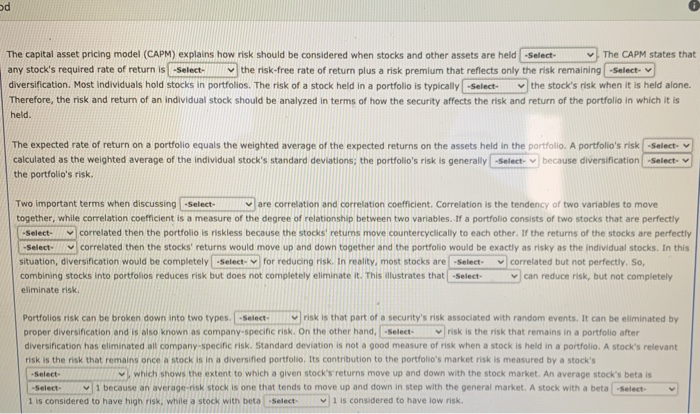od The capital asset pricing model (CAPM) explains how risk should be considered when stocks and other assets are held -Select- The CAPM states that any stock's required rate of return is -Select the risk-free rate of return plus a risk premium that reflects only the risk remaining -Select- diversification. Most individuals hold stocks in portfolios. The risk of a stock held in a portfolio is typically -Select the stock's risk when it is held alone. Therefore, the risk and...

• ### The scroll down options are 1. systematic/unsystematic risk 2. systematic/unsystematic risk 3. standard deviation/risk aversion 4....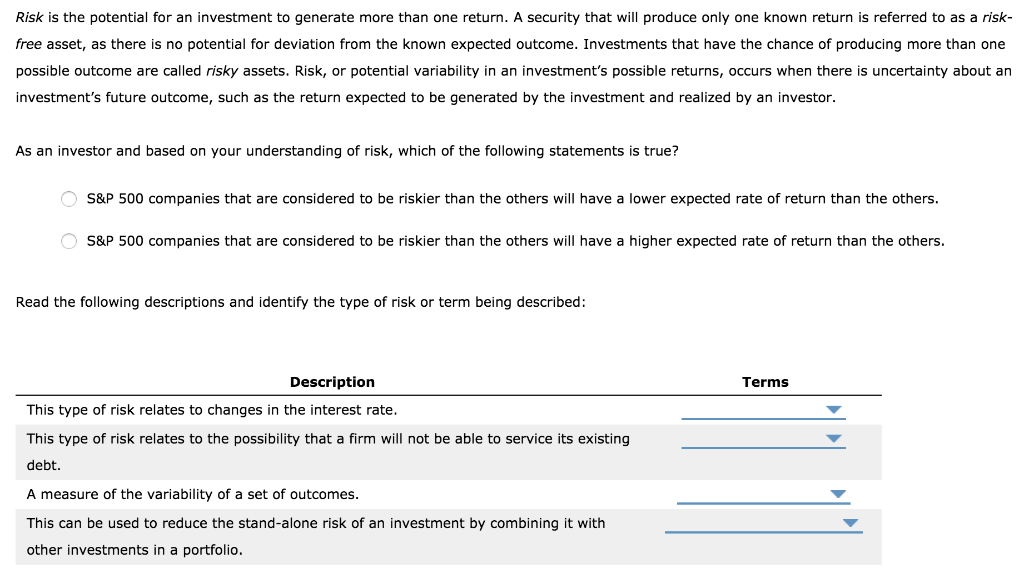The scroll down options are 1. systematic/unsystematic risk 2. systematic/unsystematic risk 3. standard deviation/risk aversion 4. correlation coefficient/diversification Risk is the potential for an investment to generate more than one return. A security that will produce only one known return is referred to as a risk- free asset, as there is no potential for deviation from the known expected outcome. Investments that have the chance of producing more than one possible outcome are called risky assets. Risk, or potential variability...

• ### p8-15 A-C 333 CHAPTER 8 Risk and Return a. If the returns of assets V and...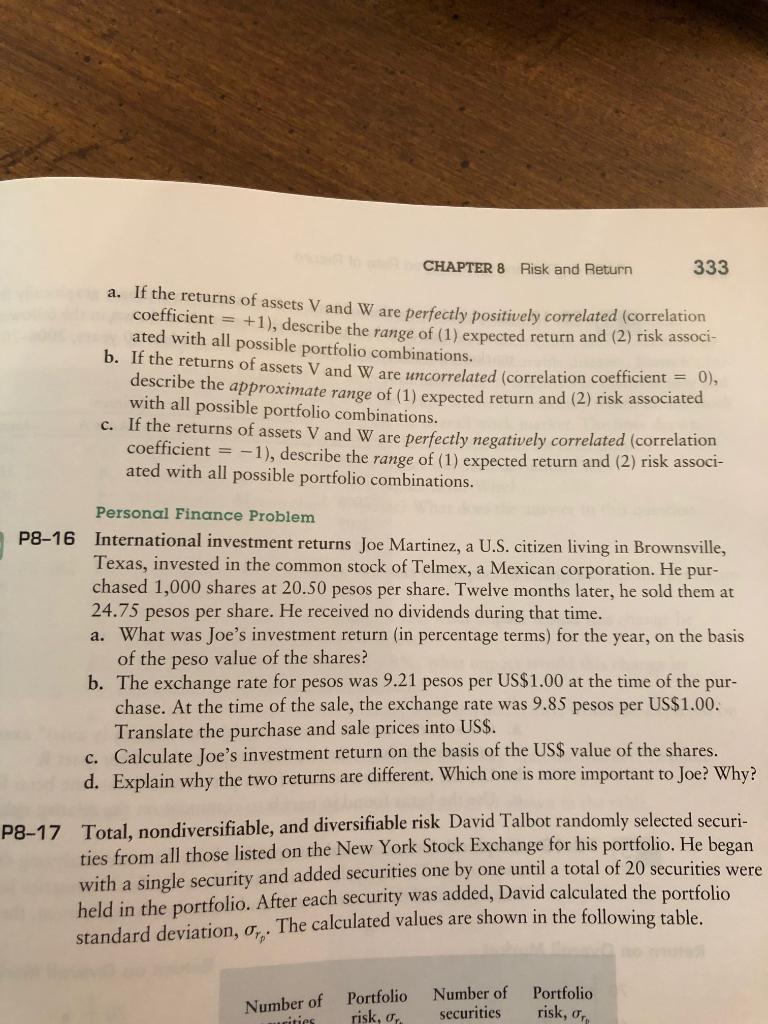p8-15 A-C 333 CHAPTER 8 Risk and Return a. If the returns of assets V and W are perfectly positively correlated (correlation coefficient = +1), describe the range of (1) expected return and (2) risk associ- ated with all possible portfolio combinations. b. If the returns of assets V and W are uncorrelated (correlation coefficient = 0), describe the approximate range of (1) expected return and (2) risk associated with all possible portfolio combinations c. If the returns of assets...

• ### A stock's contribution to the market risk of a well-diversified portfolio is called the Capital Asset...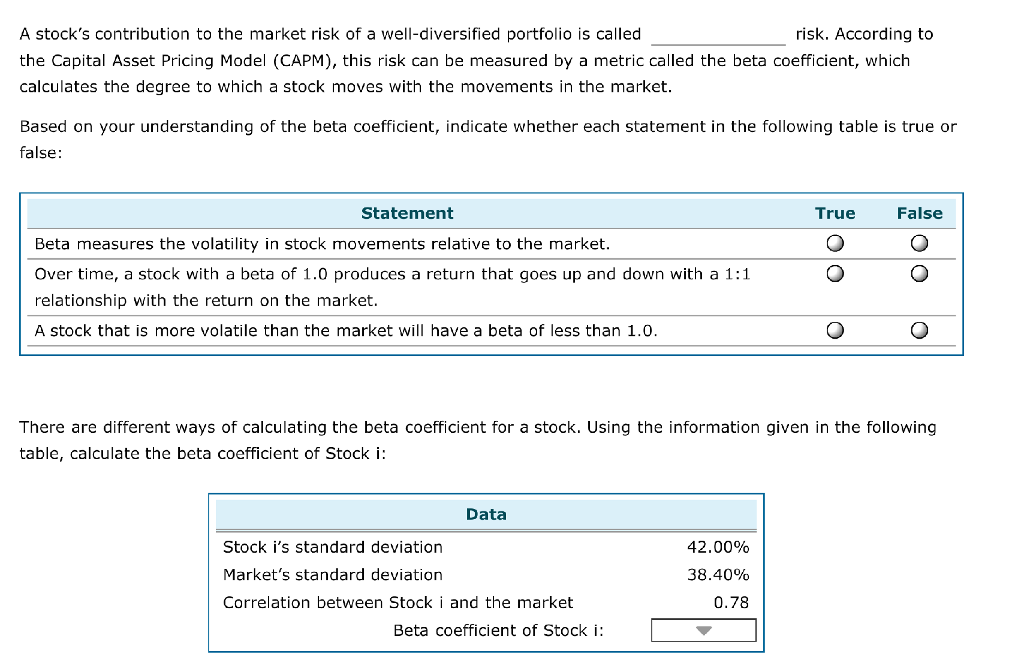A stock's contribution to the market risk of a well-diversified portfolio is called the Capital Asset Pricing Model (CAPM), this risk can be measured by a metric called the beta coefficient, which calculates the degree to which a stock moves with the movements in the market. risk. According to Based on your understanding of the beta coefficient, indicate whether each statement in the following table is true or false: Statement True False Beta measures the volatility in stock movements relative...

• ### Suppose the rate of return on short-term govemment securities (perceived to be risk free) is about...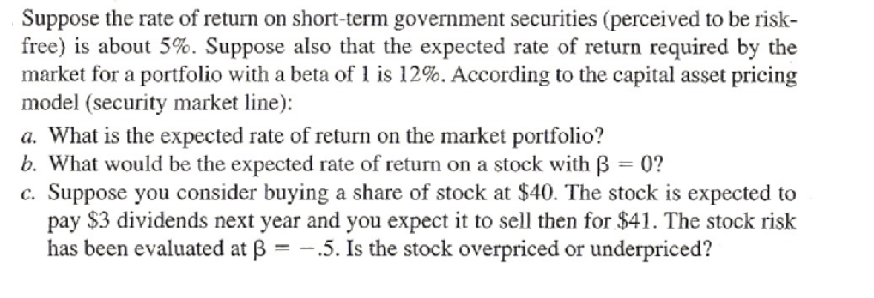Suppose the rate of return on short-term govemment securities (perceived to be risk free) is about 5%. Suppose also that the expected rate of return required by the market for a portfolio with a beta of 1 is 12%. According to the capital asset pricing model (security market line): a. What is the expected rate of return on the market portfolio? b. What would be the expected rate of return on a stock with B 0? c. Suppose you consider...